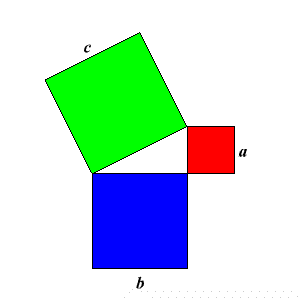# The Pythagorean Theorem

The Pythagorean Theorem is named after Pythagoras of Samos , a mathematician who was also a religious leader, and believed that all things in the universe were composed of numbers.

He is supposed to have been the first to have proved this theorem about right triangles:

Pythagorean Theorem. In a right triangle with legs of lengths $a$ and $b$ and hypotenuse of length $c$ , the following equation is true:

${c}^{2}={a}^{2}+{b}^{2}$

(There are many different ways to prove this.)

The hypotenuse of a right triangle is the side opposite the right angle.

Below is a graphical representation. The theorem states that the sum of the areas of the blue and red squares is equal to the area of the green square.Important : Remember that the Pythagorean Theorem is true only for right triangles – triangles which have a $90°$ angle.

The converse of the theorem is also true: if a triangle has sides of lengths $a,b,\text{\hspace{0.17em}}\text{\hspace{0.17em}}\text{and}\text{\hspace{0.17em}}\text{\hspace{0.17em}}c$ , and ${c}^{2}={a}^{2}+{b}^{2}$ , then it must be a right triangle .